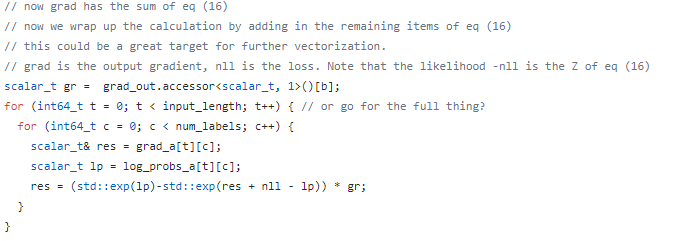# Why gradients of nn.CTCLoss is the objective function derivatives with respect to the unnormalised outputs u(t,k)

hi, I found that the gradients of nn.CTCLoss is the objective function derivatives with respect to the unnormalised outputs u(t,k), why is not the objective function derivatives with respect to the input(log probs obtained with log_softmax)? Does it compute gradients again when backpropagate through softmax layer?

thank you!

Hi,

I am not sure to grasp your question exactly.
Could you share some code that shows the mismatch you’re talking about?

thanks for your answer. The code is in pytorch/aten/src/ATen/native/LossCTC.cpp, line 300.Hi,

Thanks for the link. This should be OK no?
All these function’s gradients are checked with finite difference. So it is very unlikely that it is not computing the backward corresponding to their forward.

Hi，I can’t find the softmax op and log op in the forward functions(ctc_loss_cpu_template) in the same file, line 37. It makes me confused.

Hi,

The doc seems to indicate that it takes “Log_probs” as input. So I don’t think the log is included.
That would explain the backward formula